Next: 12.8 Proof of the Up: 12. Power Series Previous: 12.6 Trigonometric Functions   Index

# 12.7 Special Values of Trigonometric Functions

We have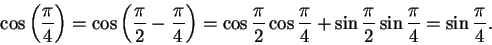Hence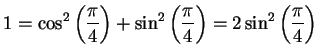, and hence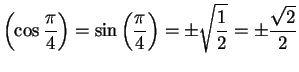. Since we knowis positive on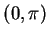, we conclude that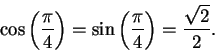Observe that if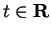, then the problem of calculating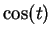and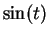is the same as the problem of calculating. Let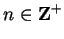. We know that the complex solutions of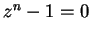are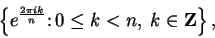so if we can express the solutions toin algebraic terms, then we can express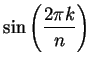andin algebraic terms. We have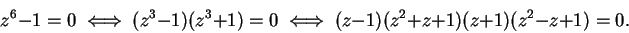Here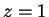andare obvious sixth roots of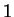, and the other four roots are the solutions of the quadratic equations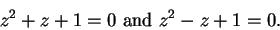12.67   Exercise. Find the solutions to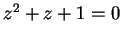and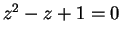in terms of square roots of rational numbers. These solutions areIdentify each solution with one of these exponentials. Find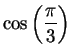and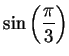.

12.68   Exercise. Use the fact that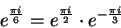to findand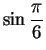.The numbers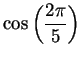and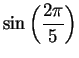can also be expressed algebraically.

If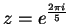, then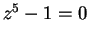, so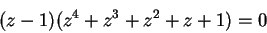and since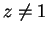,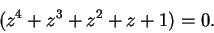The fact thatsays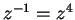and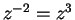, so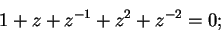i.e.,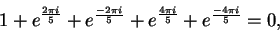or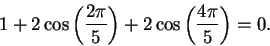Now for all,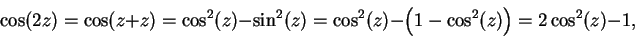so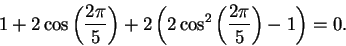(12.69)

Hencesatisfies a quadratic equation.

12.70   Exercise. A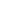a) Solve (12.69), and determineandin algebraic terms.
b) The quadratic equation has two solutions, one of which is. What is the geometrical significance of the other solution?

12.71   Entertainment. The algebraic representation forshows that a regular pentagon can be inscribed in a given circle. Let a circle be given, and call its radius. If you can constructwith compass and straightedge (see the figure), then you can construct a side of a regular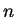-gon inscribed in the circle (and hence you can construct the-gon).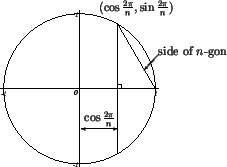For example, since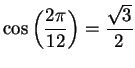, we can construct a dodecagon as follows: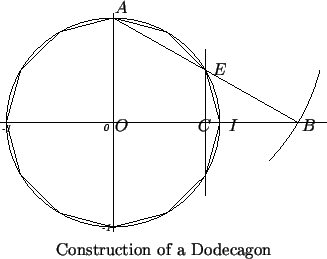In the figure, make an arc of radius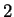with center at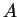, intersecting the-axis at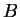. Then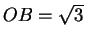, so if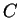bisects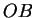, then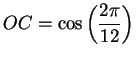, and the vertical line throughintersects the circle at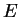whereis a side of the-gon.

Use the formula forto inscribe a regular pentagon in a circle.

12.72   Entertainment. (This problem entertained Gauss. It will probably not really entertain you, unless you are another Gauss.) Show that a regular-gon can be inscribed in a circle using compasses and straightedge.

Gauss discovered this result in 1796 [31, p 754] when he was a nineteen year old student at Göttingen. The result is [21, p 458]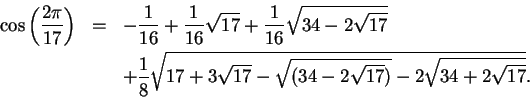Next: 12.8 Proof of the Up: 12. Power Series Previous: 12.6 Trigonometric Functions   Index